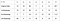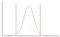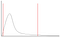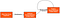Three Reasons We Bootstrap Our Experimentation Data

Bootstrapping provides numerous benefits in our experimentation analytics, but this article will cover just the three that have been most interesting to our work at Udemy. Before I get into why we use bootstrapping, I’ll give a very brief overview of the bootstrapping method. Then I’ll dive into how bootstrapping makes our computation of upper and lower bounds easier, how bootstrapping helps us with extreme values or outliers, and how bootstrapping sets us up for closed form computation of our Bayesian posteriors. Not sure what any of that means? Read on and I’ll do my best to explain each in turn.

What is Bootstrapping?

If you pull your data up by its bootstraps frequently, feel free to skip this section. If, on the other hand, you use other methods for estimating your data’s statistics, I’ll start with a brief overview of bootstrapping.

Bootstrapping is a class of methods for estimating the statistics of a set of data. In this article, when I refer to bootstrapping I’ll be referring to the simple bootstrap method. The simple bootstrap method works as follows:

1. Given a data set of size n, sample from the data set n times with replacement
2. Repeat step 1 m times (e.g., m=10,000)
3. For each vector produced by step 1, calculate the statistic of interest (e.g., the mean)
4. The result is a distribution of the statistic (e.g., if the statistic of interest is the mean, and assuming you picked the right m and n is large enough, step 3 should result in a normal distribution)Table 1. Example of bootstrapping original data and computing the mean for each bootstrap

For a visual of what this looks like when calculating means, check out the Central Limit Theorem section of Seeing Theory. Although it’s meant to demonstrate the Central Limit Theorem, the sampling method is just what you would use in simple bootstrapping.

One nice feature of using bootstrapping is that there are a few different bootstrap methods you might choose from and they can be swapped in and out without affecting other models in your statistical analysis. Take our analysis pipeline (Figure 1), where you can see that swapping in a different bootstrapping method will not require any changes to the rest of the models in our pipeline. Concretely, we use simple bootstrapping, which is equivalent to Bayesian bootstrapping with a uniform prior. But suppose we found that a non-uniform prior for the bootstrap method is preferable. For instance, we might want to have less probability that an outlier is sampled relative to other sample units. If we thought that, we could swap out the simple bootstrapping method with an explicit Bayesian bootstrapping method in our analysis pipeline and it would simply work for both our primary experiment metric and our exploratory analysis metrics.

For a detailed overview of simple bootstrapping and some alternatives, see Efron (1977). For a less detailed overview, see Wikipedia’s entry on bootstrapping.

Credible Intervals

Credible intervals are often confused with what frequentists call confidence intervals, but credible intervals are measures of uncertainty in your belief about the data, e.g., a 95% credible interval means that you should be 95% confident (i.e., have a credence of .95) that the data falls in the interval. One type of credible interval is the highest density interval (HDI), which is the interval of the distribution that contains the highest density (see Figure 2).Figure 2a. Example of the highest density interval (HDI) for a symmetrical distributionFigure 2b. Example of the highest density interval (HDI) for a right skewed distribution

Oftentimes experiment decisions are reported as point estimates. But it’s easy to get the same point estimates with statistical significance from experiments that have very different value to the organization. For instance, experiments whose data has very different variance can have the same point estimate, but the experiment with more variance can be more risky. Thus, point estimates lose important information with regard to the value of an experiment. So, one of the statistics we want to use to make and report decisions is the 95% upper and lower bounds of the HDI. In addition, we compute this for many different metrics: everything from impressions to revenue. Unfortunately for many distributions, there is no closed form function for computing the HDI.

However, since what we really care about is the sampling distribution of the means, we only need to worry about computing the HDI for that distribution, viz., the normal distribution. Bootstrapping allows us to use the same approach for computing HDIs without having to worry about the underlying distribution because the bootstrapped distribution is a normal distribution. We could have as the underlying distribution a binomial distribution, a multimodal distribution, or any other type of distribution, and we can compute the HDI of the sampling distribution using the same method.

For symmetrical distributions like the normal, the HDI is the same as the central interval, e.g., the 95% HDI is the 95% central interval of a symmetric distribution. This allows for a simple method for computing the HDI, the basic percentile method. However, it’s not an option if your posterior is the parameters of a normal distribution because the basic percentile method is computed via the units of the samples, and after a Bayesian update we only have the parameters of the posterior distribution. However, the basic percentile method is fairly efficient and worth noting. You merely take the value at the (100%-α)/2 and α+(100%-α)/2 as the lower and upper bounds, respectively. For example, if you want a 95% lower bound you use (100%-95)/2, which is 2.5%, and find the value at n*.025. That is, if n=1,000, you take the value at position 25 in the ascending ordered collection of values, and that is your lower bound (mutatis mutandis for the upper bound).

If, however, you’re using bootstrapping as the likelihood in a Bayesian update you will need another method. Given that the posterior is the parameters of a normal distribution though, this means we have a closed form method for computing the HDI of our posterior. If the distribution we were trying to compute the HDI for was not symmetrical, we would likely have to use a brute force method to find the interval.

Extreme Values

With traditional experimentation analysis, like t-tests, outliers can cause a big problem. Here at Udemy, we had an experiment where one outlier in the sample resulted in a significant experiment showing up as not significant. The lesson learned is that even a single, large outlier can cause your experiment to be called incorrectly for certain metrics. One way to solve this issue is to remove outliers altogether, but outliers are legitimate data points and you will need to draw some arbitrary cutoff point for when to exclude them. This could result in significant bias in your analysis.

Consider bootstrapping a sample n=one million units. If there is one large outlier, the chance it will be sampled is one in a million for each unit sampled, or a 63% chance it is sampled at least once for each bootstrap sample of size n. So, for m=10,000 bootstrap samples of size n, we should expect that only 6,300 of those include the outlier.

The upshot is that you do not have to arbitrarily remove data from your underlying distribution, because the distribution of the means should better reflect the unlikeliness of the outlier than the original sample does.

Consider this example:

```library(boot)set.seed(187)n <- 10000
mu <- 1
sigma <- 1
control <- rnorm(n, mu, sigma)
variant <- rnorm(n, mu * 1.05, sigma)print("Without Outlier")
t.test(control, variant)print("With Outlier")
control <- mu + sigma * 200
t.test(control, variant)print("Outlier With Bootstrapping")bootpothesis <- function(x, y, bs_n) {
sample_tstat <- abs(t.test(x, y)\$statistic)pooled_mean <- mean(c(x,y))
x1 <- x-mean(x) + pooled_mean
y1 <- y-mean(y) + pooled_meanbootstrapped_tstats <- vector(length = bs_n)
for (i in 1:bs_n) {
boot_control <- sample(x1, size=length(x), replace=T)
boot_variant <- sample(y1, size=length(y), replace=T)
bootstrapped_tstats[i] <- t.test(boot_control, boot_variant, var.equal=TRUE)\$statistic
}return(mean(bootstrapped_tstats > sample_tstat))
}bs_n <- 10000bootpothesis(control, variant, bs_n = bs_n)```

Without the outlier, you see a p-value of 0.0005167; with the outlier, it’s 0.2421. However, when we use a bootstrap hypothesis test with the outlier, we get a much more reasonable p-value of 0.0723. Note that I’m using bootstrap hypothesis just for illustration purposes; we do not use bootstrap hypothesis testing or any other form of null hypothesis testing at Udemy. But the p-values in the example do a good job of showing where the means fall with respect to the distribution’s confidence interval. When we ran into this problem previously we were using t-tests.

Another way you could handle outliers is by using Bayesian bootstrapping, where you specify ahead of time some prior probability distribution for your bootstrapped data. Then, if your outlier falls in an area of the distribution with low probability, the posterior will reflect that and the outlier will be given less weight.

Normal Distributions

I mentioned that normal distributions would make HDI computation a piece of cake, but normal distributions are useful for other reasons. Specifically, for our experimentation analysis we use Bayesian statistics for computing the posterior probability distribution of the treatment effect for each variation. We also use Bayesian statistics for our exploratory dashboard for gaining insights after an experiment has run. Again, if we had to handle every distribution type for the metrics on these dashboards, we would be doing a lot of work.

With bootstrapping we always get a normal distribution of means. Combine this with the fact that normal distributions have analytic, conjugate priors, the result is that our work is generalizable and much simpler. Conjugate priors provide us closed form solutions for updating our posteriors, which means the computation is extremely fast. In addition, the posterior and the prior have the same form, in our case the Normal Inverse Gamma, which means our posterior is a natural prior for the next batch of experiment data we process. That is, in Figure 1 above you could draw a line from the posterior back to the point where the likelihood is combined with the prior (Figure 3). This allows our posterior to be continually updated as new data comes in.Figure 3. The posterior becomes the prior after the first Bayesian update

Recap

I briefly covered three reasons we use bootstrapping. First, it allows us to compute credible intervals for the sampling distribution consistently regardless of the underlying distribution. Second, it allows us to handle outliers without arbitrary cutoffs. We can either rely on the unlikeliness of the outlier being sampled or we could use Bayesian bootstrapping to weight the outlier lower than other values without excluding it altogether. The third benefit is that we can use Bayesian statistics in a computationally efficient manner because normal distributions, which bootstrapping provides us when targeting the means, have conjugate priors.

Bootstrapping provides other benefits, but there are also trade-offs. For example, bootstrapping is computationally expensive. However, there are methods for making bootstrapping less expensive, and we will cover one of those in our next post: Bootstrapping with Spark.

If you have questions about anything covered in this post, please leave a comment, and we’ll do our best to provide an answer. If these sound like interesting problems to work on, please check Udemy’s Careers page. We’re always looking for inquisitive people to work with.

Written by